Vibroengineering PROCEDIA

Published: 19 October 2018

# Gear pitting level diagnosis using vibration signals with an improved inception structure

Xueyi Li1
Xu Li2
Yongzhi Qu3
David He4
1, 2, 4School of Mechanical Engineering and Automation, Northeastern University, Shenyang, Liaoning, China
3School of Mechanical and Electronic Engineering, Wuhan University of Technology, Wuhan, Hubei, China
Corresponding Author:
David He
Views 152

#### Abstract

Gear pitting fault is common in mechanical devices. At present, most of the gear pitting fault detection methods are based on the manual extraction of the frequency domain features from vibration signals. This paper presents a method for gear pitting fault level diagnosis using vibration signals with an improved inception network. The presented method directly applies to the vibration signals to automatically extract features and diagnose the level of the gear pitting fault using deep learning. The presented method has been validated with vibration data collected for 7 gear pitting conditions from gear pitting fault tests. The validation results have shown that the presented method can effectively classify the levels of the gear pitting faults. In comparison with traditional convolutional neural network, the diagnosis accuracy has been significantly improved with the presented method.

## 1. Introduction

Gearboxes are a common transmission component in industrial machines. Gearpitting is one of the most common faults of a gearbox. The gear pitting fault level diagnosis would have a significant impact on the reduction of the maintenance costs.

The traditional gear fault detection method mainly relies on the manual extraction of features to train the network. A new narrowband interference cancellation method was used to suppress the narrowband component and enhance the pulse component, making gear fault detection easier . By sorting effectiveness scores, Liu et al.  proposed criterion was validated by the University of California Irvine benchmark datasets and are also applicable to the pitting diagnosis of planetary gearboxes. Although manual extraction features can make a diagnosis, a large amount of prior knowledge was required as a basis. Moorthy et al.  observed that micro-cracks tend to take precedence over the micro-valley on the surface of the ground gear against the sliding direction, especially when the depth of the micro-valley was advantageously oriented at an angle relative to the sliding direction. Many detection methods used frequency domain signals for training. Feng et al.  observed that the amplitude envelope and instantaneous frequency of the planetary gearbox vibration signal were related to the characteristic frequency of the faulty gear, a joint amplitude, and frequency demodulation method was presented for the fault diagnosis of the planetary gearbox. Although the frequency domain signal can better reflect the pitting fault characteristics of the gear, the conversion from the time domain to the frequency domain requires additional time and computational cost.

With the advancement of technology and the improvement of computing power, more and more methods were used in the diagnosis of gear pitting faults. Morsy et al.  applied continuous wavelet transform, fast Fourier transform algorithm, and order analysis measurements to detect artificial pitting defects in the gear by tracking acceleration and gearbox response under different loads. Qu et al.  presented unsupervised sparse autoencoder combined with dictionary learning for fault diagnosis of gear pitting.

The main content of the paper is arranged as follows. Section 2 elaborates on the modified inception algorithm. In Section 3, the description of the gear seeded pitting fault test experiment used to validate the presented method is provided. Section 4 analyzes and discusses the experimental results. Section 5 summarizes the paper.

## 2. The methodology

The method uses a modified inception structure. The main idea of the inception architecture is to solve the problem of how to use a simple and achievable dense substructure to approximate and replace an optimal local sparse structure in a convolutional network . The inception structure is composed of a convolutional neural network (CNN).

A CNN consists of an input layer, an output layer, and several hidden layers. The types of hidden layers of the CNN include a convolutional layer, a pooling layer, and a fully connected layer. The physical meaning of the convolutional layer in CNN is extracting the characteristics of the local domain. The output of the convolver is calculated by the following formula:

1
${C}_{i,k}=\theta \left({\sum }_{b=1}^{s-1}{w}_{b,k}{v}_{b+i}^{T}+{a}_{k}\right).$

In Eq. (1), $k$ represents the $k$th convolver, ${v}_{b+i}^{T}$ the $i$th input eigenvector, ${w}_{b,k}$ the weight parameter of the $k$th convolver, $s$ the width of the convolver, and ${a}_{k}$ the network bias. After weighted averaging of the $i$th input and the $k$th convolver, an output node value of the convolutional layer will be obtained by the nonlinear function $\theta$. $\theta$ is generally selected as an inverse tangent function or a sigmoid function.

Fig. 1The general procedure of the presented method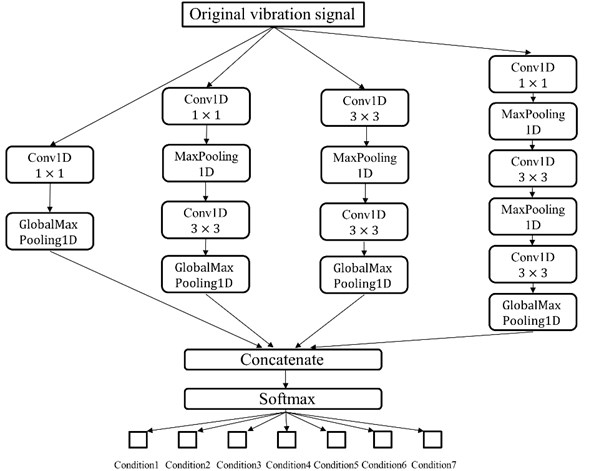The traditional inception module is the simplest design of the inception structure. Its output is a combination of three convolutional layers and one pooled layer. The disadvantage of this design is that the pooling layer does not change the number of channels of the feature. After splicing, the number of channels of the feature is large. After several layers of modules are stacked, the number of channels will become larger. So, will the number of the parameters. To overcome this shortcoming, the improved inception model introduces three 1×1 convolutional layers to reduce the number of channels as the 1×1 convolutional layer can correct linear features. Because all the convolution kernels can work on all outputs after the previous layer, the 5×5 convolution kernel would require too much computational effort. The method presented in this paper limits the size of the convolution kernel to 3×3 so that the computational effort will be reduced to some extent. As shown in Fig. 1. The method presented in this paper adopts 4 parallel neural networks to train at the same time. The network of each branch contains the convolutional layer and the pooling layer. The trained network finally performs the diagnosis of the gear pitting fault levels in the softmax layer. Since the number of layers of the neural network is relatively small, the gradient back-off phenomenon is not obvious. The network design uses only one softmax layer and one loss function, thereby reducing network complexity and improving its computing performance.

## 3. The experiment setup

The gear pitting fault diagnosis experiment was carried out in a gearbox test rig as Fig. 2. It consisted of two 45 kW Siemens servo motors, one of which acted as a drive motor and the other could be configured as a load motor. The test gearbox is a single stage spur gearbox. The main gear parameters are shown in Table 1.

Fig. 2The gearbox test rid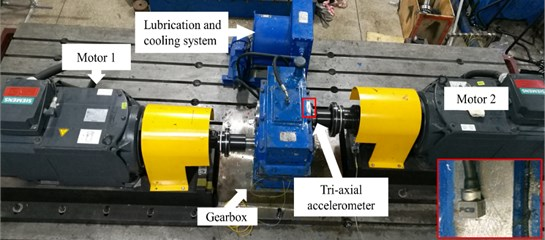Table 1The primary parameters of the gearbox rig

 Gear parameter Driving gear Driving gear Tooth number 72 40 Module (mm) 3 3 Pitch diameter (mm) 120 120 Base circle diameter (mm) 202.974 112.763 Pressure angle (°) 20 20 Tooth width (mm) 85 85

The 7 gear pitting conditions are shown in Table 3. Condition 1 is a normal gear without pitting. From Condition 2 to Condition 4, the middle gear had 10 %, 30 %, and 50 % pitting respectively. In Condition 5, the intermediate gear teeth had 50 % pitting and 10 % pitting on the adjacent upper tooth. In Condition 6, the intermediate gear tooth had 50 % pitting and the adjacent upper and lower tooth both had 10 % pitting. In Condition 7, the intermediate gear teeth had 50 % pitting, 30 % pitting on the upper tooth, and 10 % wear on the upper teeth. The pitting conditions of the 6 gears are shown in Fig. 3.

The sample vibration signals of the 7 pitting gears were shown in Fig. 4. The gears were mounted symmetrically, so the radial is the main through vibration direction. Furthermore, the stiffness of the gearbox along the vertical direction to the ground is smaller than the other axes. In this paper, the $z$-axis signals were used for analysis. The sampling length of signal is decided by the rotating speed to ensure the gear revolution information included in each sample.

The data were processed and analyzed on Windows 10 operating system with Intel Core i5-6500 processor, NVIDIA GTX 1080Ti graphics card, and Kingston 16GB memory. The software used in the experiment include MatLab R2018a, Python 3.6, CUDA 8.0, and cudnn 9.2.

Table 2The approximate percentage of pitting area under seven gear conditions

 Gear condition Upper tooth Middle tooth Lower tooth Condition1 Normal Normal Normal Condition2 Normal 10 % Normal Condition3 Normal 30 % Normal Condition4 Normal 50 % Normal Condition5 10 % 50 % Normal Condition6 10 % 50 % 10 % Condition7 30 % 50 % 10 %

Fig. 3Pitting degree of driven gears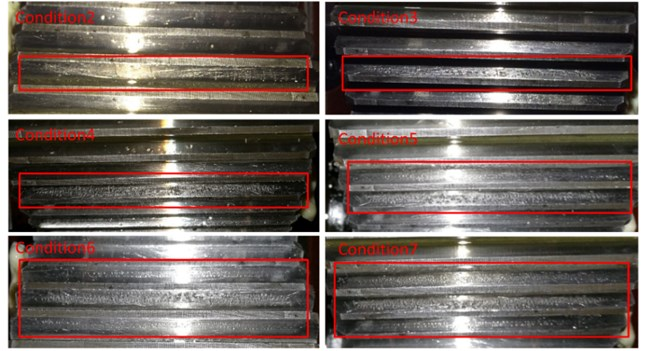Fig. 4Raw vibrational signals of gear pitting fault conditions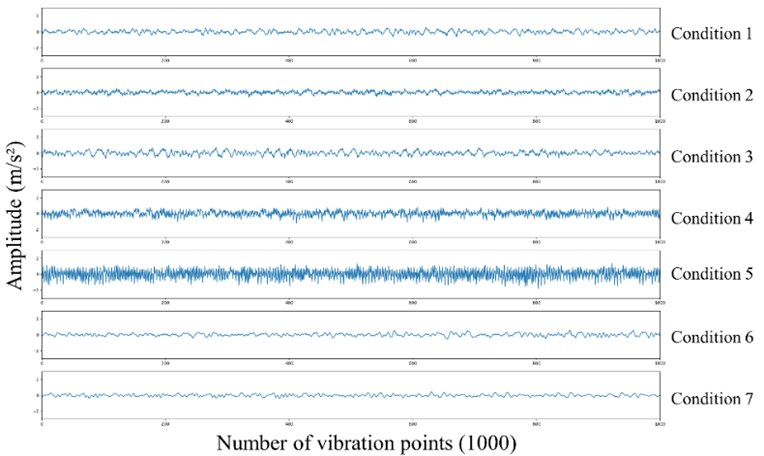## 4. The results and discussions

The gear raw vibration data in the experiment was used for training. The results are shown in Table 3. The method proposed in this paper has a significant improvement on the detection of gear pitting failure compared with the application of convolutional neural network only. The accuracy of the testing set can be reached. 98.9 %, while CNN can just reach 73 %. The method presented in this paper can effectively avoid the over-fitting problem generated in the deep learning process. For the diagnosis results of each type of gear pitting failure, as shown in Table 4, it can be seen that five types of gears have been correctly diagnosed, and the other diagnosis results of the two kinds of pitting wear gears are all above 95 %. It embodies the effectiveness of the presented method for gear wear fault detection.

The accuracy curve and loss curve of the presented method are shown in Fig. 5. It can be seen from the figure that the accuracy of the model is stable after 150 epochs, and there is no obvious inflection point in the curve. The confusion matrix results of this test set are shown in Fig. 6. As can be seen from the figure, for Condition 2, a pitting gear was misidentified into the Condition 4. For Condition 3, two pitting gears were misidentified into the Condition 2. It can be seen from Fig. 3 that the gears of these three states are indeed very close, considering that the vibration signal of the gearbox is also affected by the external environment.

The classification result of the training set used t-SNE to reduction dimensionality. As shown in Fig. 7, it can be seen from the figure that the gears of the seven pitting conditions are clustered. There were having obvious boundaries and almost no coincidence, indicating that the method proposed in this paper has a good classification effect on the wear fault detection of gears.

Fig. 5The comparison of accuracy and loss between the training set and the validation set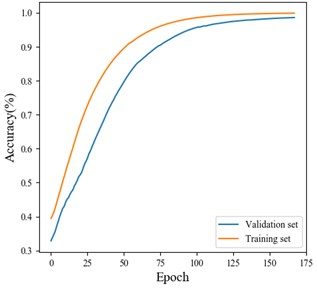a) Accuracy comparison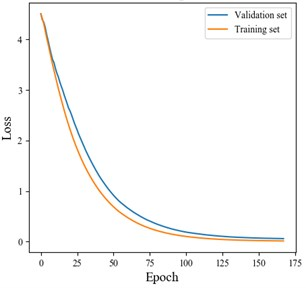b) Loss comparison

Fig. 6The confusion matrix by the presented algorithm for the testing set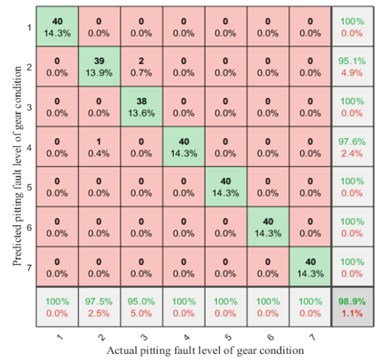Fig. 7The visualization of three-dimensional features of gear pitting conditions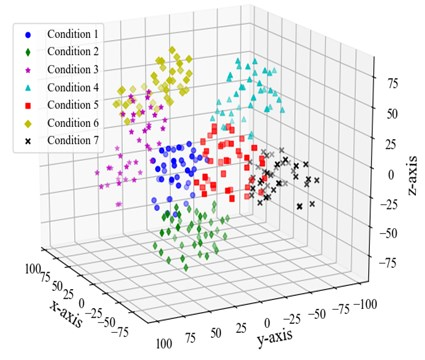Table 3The accuracy comparison between the presented algorithm and the CNN

 Model Training set Validation set Testing set Inception 100.00 % 98.93 % 98.90 % CNN alone 98.16 % 80.00 % 73.57 %

Table 4Classification accuracy results of the testing samples

 Model The accuracy of seven pitting conditions Total 1 2 3 4 5 6 7 Inception 100 % 97.5 % 95 % 100 % 100 % 100 % 100 % 98.9 %

## 5. Conclusions

This paper presented a method for diagnosis of gear pitting fault level using vibration signals. The presented method uses a deep learning method based on an improved inception structure to automatically extract features from vibration signals and diagnose gear pitting faults. The effectiveness of the method was validated with data collected from seeded gear pitting fault tests in a gearbox test rig in the laboratory. The validation results have shown that the presented method can effectively detect gear pitting fault levels.

#### Cited by

International Conference on Engineering Tribology and Applied Technology
A comprehensive review on convolutional neural network in machine fault diagnosis